×
Get Full Access to Introductory Chemistry - 5 Edition - Chapter 10 - Problem 75p
Get Full Access to Introductory Chemistry - 5 Edition - Chapter 10 - Problem 75p

×

# Determine the molecular geometry of each polyatomic ion.(a) CO32?(b) ClO2?(c) NO3?(d)ISBN: 9780321910295 34

## Solution for problem 75P Chapter 10

Introductory Chemistry | 5th Edition

• Textbook Solutions
• 2901 Step-by-step solutions solved by professors and subject experts
• Get 24/7 help from StudySoup virtual teaching assistantsIntroductory Chemistry | 5th Edition

4 5 1 358 Reviews
22
5
Problem 75P

PROBLEM 75P

Determine the molecular geometry of each polyatomic ion.

(a) CO32−

(b) ClO2−

(c) NO3−

(d) NH4+

Step-by-Step Solution:

Problem 75P

Determine the molecular geometry of each polyatomic ion.

(a)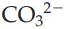(b)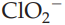(c)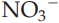(d)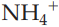Step by Step Solution

Step 1 of 4

The three dimensional arrangement of atoms in a molecule is called molecular geometry.

Number of electrons around the central atom in its Lewis structure helps to predict the geometry of the molecule or ion.

Geometry of the ion or molecule is mainly predicted by the number of lone pairs and bond pairs around the atom.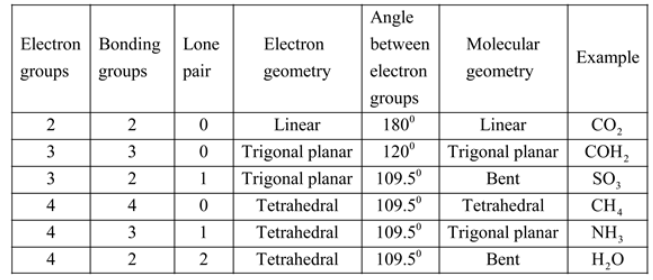The given ion -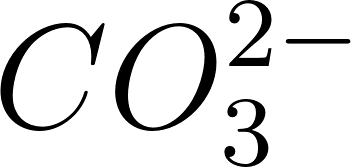.

The structure of the given ion is as follows.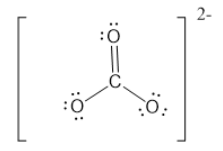The central atom's carbon and it has 3 electron groups all are bonding.

Hence, central atoms have 3 electron groups and 3 bonding pairs.

Therefore, geometry of the molecule is trigonal planar.

____________________________________________________________________________

Step 2 of 4

Step 3 of 4

##### ISBN: 9780321910295

Unlock Textbook Solution